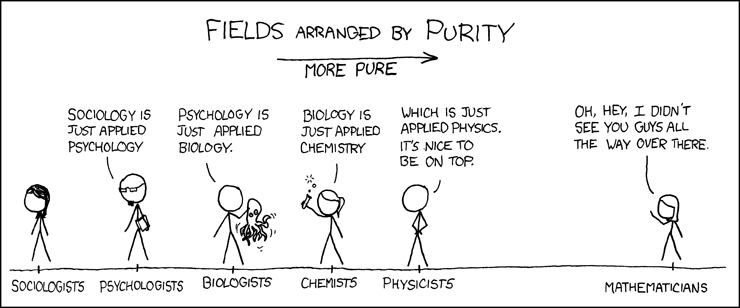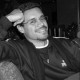You are currently browsing the monthly archive for December 2011.

What if mathematical theories could be expressed using artworks instead of plus signs and pi symbols? Dorothea Rockburne has devoted four decades to the attempt. View PBS’s Sunday Arts Profile on Rockburne on YouTube.

Also, on most YouTube videos, the player has a six-corner snowflake (next to CC) that gives you an option to view various snowflake configurations falling throughout the video. YouTube has placed the temporary six-cornered snowflake button for the holidays. I think it is highly fitting given the post below “The Six-Cornered Snowflake of Kepler”!

View all her works here.

The use of mathematics to read the book of nature.

Capi Corrales Rodrigáñez
Department of Algebra, Faculty of Mathematics, Complutense University of Madrid, Madrid, Spain

Abstract. “Philosophy is written in that great book which ever lies before our eyes – I mean the universe – but we cannot understand it if we do not first learn the language and grasp the symbols, in which it is written. This book is written in the mathematical language, and the symbols are triangles, circles and other geometric figures, without whose help it is impossible to comprehend a single word of it; without which one wanders in vain through a dark labyrinth,” wrote Galileo (Il Saggiatore, chapter 6, p. 4). In 1611, the mathematician Johannes Kepler, a contemporary of Galileo and voracious reader of the book of the world, wrote his shortest and most surprising book, The Six-Cornered Snowflake: A New Year’s Gift. “Even as I write these things, it has begun to snow again, and more thickly than before. I have been attentively observing the tiny particles of snow, and although they were all falling with pointed radii, they were of two kinds. Some were exceedingly small, with varying numbers of radii that spread in every direction and were plain, without tufts or striations. These were most delicate, but at the same time joined together at the center in a somewhat larger droplet; and they were the majority. Sprinkled among them were the rarer, six-cornered snowflakes” (Kepler, 1611). This text by Kepler, little known outside the physics and mathematics community, marked a milestone in the use of mathematics to understand a part of the physical world that surrounds us. With this text as a map, this article covers part of the terrain explored by geometry, from the 3rd century AD until today.

Capi Corrales Rodrigáñez is professor of the Department of Algebra of the Faculty of Mathematics of the Complutense University of Madrid. She obtained her Ph.D. at the University of Michigan (USA), where she specialized in the theory of algebraic numbers. She combines research with the scientific popularization of contemporary mathematics.

Fancy some Christmas ornaments that defy conventional Euclidean geometry? In these animations, created by mathematician and artist Jos Leys, you can see what Christmas ball patterns would look like in hyperbolic space, a “bizarro world” where parallel lines can intersect and the three angles of a triangle add up to less than 180 degrees.

The different ornament designs show various ways that 2D hyperbolic space can be tiled with polyhedron shapes by varying the Poincaré disc model. Developed by Henri Poincaré of Poincaré conjecture fame these tesselations have also been explored by artist MC Escher in a series of prints.

For more mathematical imagery, check out a gallery of art in the hyperbolic realm, hyperbolic home decoration or visit Leys’ web site. You may also like to watch our series of One-Minute Math animations.

View video here.“A team of mathematicians from the University of Vermont analyzed 4.6 billion Twitter messages worldwide over 33 months. They assigned happiness grades to more than 10,000 of the most common words, crunched all the numbers and plotted them on a graph that shows a gradual downward slope during the past year and a half or so, through mid-September.” Read the article here.

Proofs from THE BOOK by Martin Aigner and Günter Ziegler begins by giving six proofs of the infinity of primes. We will go over the second proof. Before we go over this proof, lets cover some background.

— 1. Algebraic Structures —

One of the themes of modern algebra is to compare algebraic structures. An algebraic structure refers to a nonempty set equipped with a binary operation or several binary operations (usually two). Also, we shall refer to a nonempty set equipped with two binary operations satisfying certain properties as a number system. First, we shall define algebraic structures called group, ring and field. A group consists of nonempty set with one binary operation satisfying several conditions. It is the simplest of the three.

Definition 1 Let${G}$ be a nonempty set and${*:G\times G\rightarrow G}$ be a binary operation on${G.}$ Then the set${G}$ together with the binary operation${*}$, denoted${(G,*)}$, is a group, if the following three conditions are satisfied:

i. The binary operation${*}$ is associative, i.e.,${(a*b)*c=a*(b*c)}$ for all${a,b,c\in G}$.

ii. There is a${*}$-identity${e\in G}$, i.e.,${e*x=x*e=x}$ for all${x\in G}$.

iii. For each${a\in G}$, there is a${*}$-inverse${a'\in G}$, i.e.,${a*a'=a'*a=e}$, where${e}$ is the${*}$-identity.

Further more, a group${(G,*)}$ is said to be commutative (or abelian), if the binary operation${*}$ is commutative.

I have re-posted this to test my changes to the latex2wp.py and terrystyle.py programs, compiled with Python Software Foundation’s Python 2.7.2 64 bit version, to add support for LyX 1.6.9. The code change incorporates some additional theorem-like environments, macros, font styles, and the numbering has been change so that the different theorem-like types each have a separate counter (e.g., theorem 1, theorem 2, lemma 1, proposition 1, theorem 3, lemma 2, …, as opposed to theorem 1, theorem 2, lemma 3, proposition 4, …). Furthermore, I have provided more background information which will benefit those without a background in abstract algebra.

Proofs from THE BOOK by Martin Aigner and Günter Ziegler begins by giving six proofs of the infinity of primes. The first proof is what they call "the oldest Book Proof" attributed to Euclid. Before we go over this proof, lets cover some background. Read the rest of this entry »

Proofs from THE BOOK by Martin Aigner and Günter Ziegler begins by giving six proofs of the infinity of primes. The first proof is what they call “the oldest Book Proof” attributed to Euclid. Before we go over this proof, lets cover some background. Read the rest of this entry »